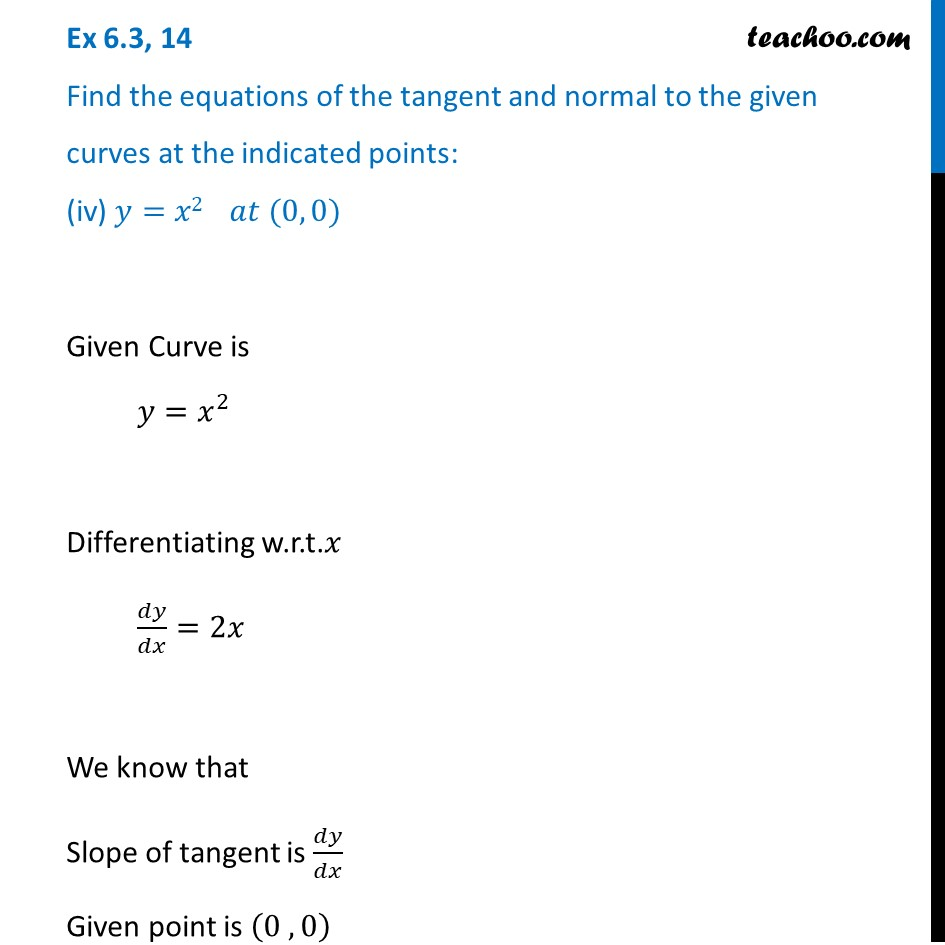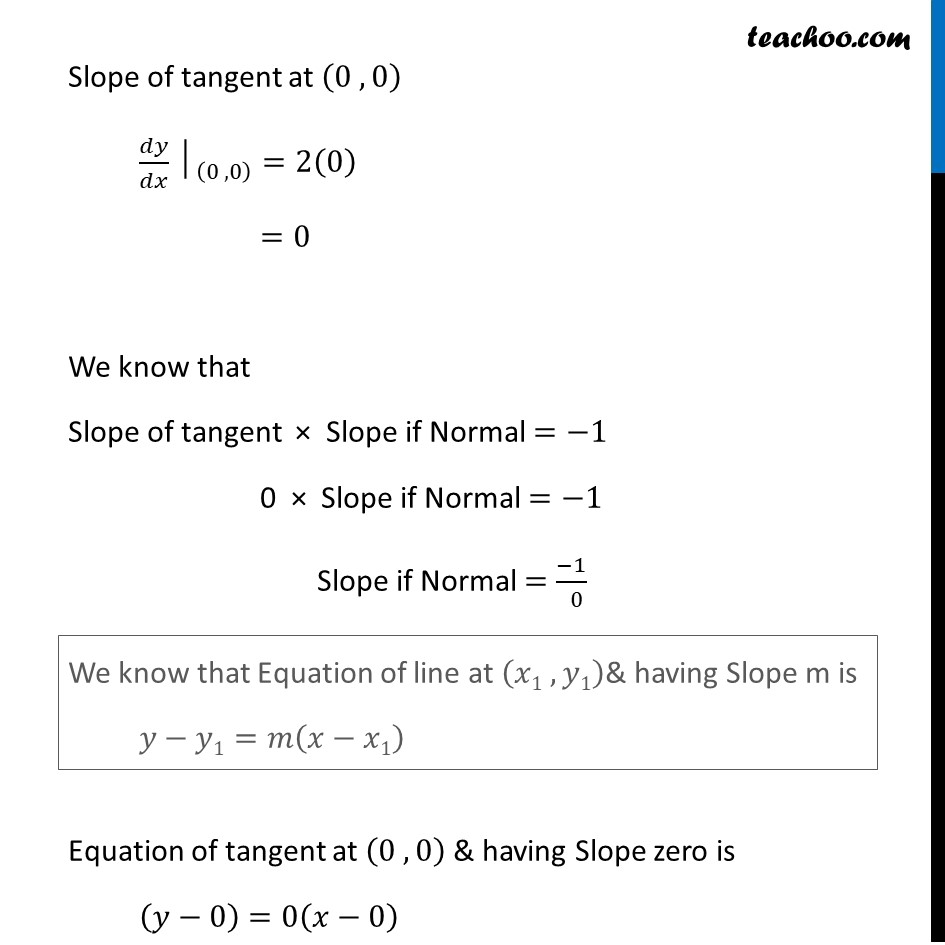Tangents and Normals (using Differentiation)

Chapter 6 Class 12 Application of Derivatives
Serial order wiseLearn in your speed, with individual attention - Teachoo Maths 1-on-1 Class

### Transcript

Question 14 Find the equations of the tangent and normal to the given curves at the indicated points: (iv) 𝑦=𝑥2 𝑎𝑡 (0, 0) Given Curve is 𝑦=𝑥^2 Differentiating w.r.t.𝑥 𝑑𝑦/𝑑𝑥=2𝑥 We know that Slope of tangent is 𝑑𝑦/𝑑𝑥 Given point is (0 , 0) Slope of tangent at (0 , 0) 〖𝑑𝑦/𝑑𝑥│〗_((0 , 0) )=2(0) =0 We know that Slope of tangent × Slope if Normal =−1 0 × Slope if Normal =−1 Slope if Normal =(−1)/( 0) Equation of tangent at (0 , 0) & having Slope zero is (𝑦−0)=0(𝑥−0) We know that Equation of line at (𝑥1 , 𝑦1)& having Slope m is 𝑦−𝑦1=𝑚(𝑥−𝑥1) Finding equation of tangent & normal Now Equation of line at (𝑥1 , 𝑦1) & having Slope m is 𝑦−𝑦1=𝑚(𝑥−𝑥1) Equation of tangent at (0, 0) & Slope 0 is (𝑦−0)=0(𝑥−0) 𝑦−0=0 𝒚=𝟎 Equation of Normal at (0, 0) & Slope (−1)/0 is (𝑦−0)=1/0 (𝑥−0) 0 × (𝑦−0)=1(𝑥−0) 0=𝑥−0 𝒙=𝟎